• A+

# A Weighting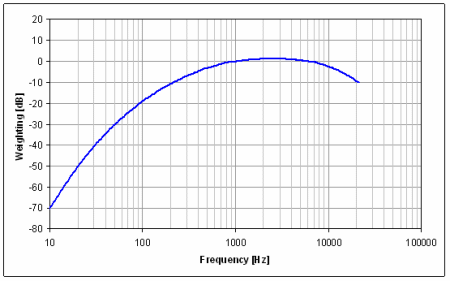This is the most generally used filter when making overall noise measurements. The attenuation of the sound signal with an A-weighted filter corresponds to the fact that the human ear is not as sensitive to sound of the lower frequencies as it is at the higher frequencies.

The A-Weighted Sound Level is a single number measure of the relative loudness of noise that is used extensively in outdoor environmental noise standards. The ratings correlate well with human judgments of relative loudness, but do not take into account the spectral balance or sound quality. Many different sounding spectra can result in the same numeric value, but have quite different subjective qualities. The A-Weighted Sound Level can be measured with simple sound level meters; the rating is expressed as a number followed by dB(A) or dBA. For example, 35dB(A).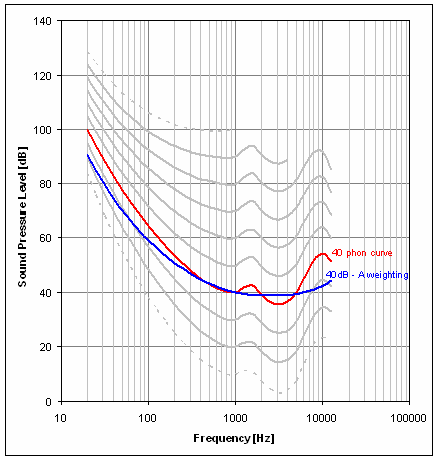The A weighting curve approximately follows the equal loudness curve of 40 phons.

The 40 phon curve shown in red and the inverted (40dB-A weighting) A-weighting curve shown in blue.

The inverted A weighting curve was calculated based on the fact that the A-weighting is 0dB at 1000Hz and the 40 phon curve is 40dB at 1000Hz, therefore, these two points coincide.

A-weighted levels are not a suitable descriptor for low frequency noise 20 to 200Hz. A-weighted sound pressure level has been found to be an unsatisfactory descriptor to predict effects caused by low frequency noise. According to previous studies, specific sound characteristics that are not fully assessed by an A-weighted sound pressure level could be of importance for adverse effects of low frequency noise.

The A-weighting value in decibels as a funcion of frequency is given by: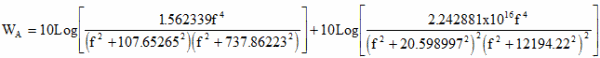where

WA = weighting to be applied, dB
f = frequency, Hz
Enter frequency and level in either dB or dB(A) to get conversion and weighting or frequency only to get weighting.
 Frequency Hz Level dB Level dB(A) A weighting dB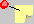The following are tabulated standard A weighting values for the 1/3 Octave centre frequencies.

 Frequency Hz A weighting dB Frequency Hz A weighting dB 20 -50.5 800 -0.8 25 -44.7 1000 0 31.5 -39.4 1250 0.6 40 -34.6 1600 1 50 -30.2 2000 1.2 63 -26.2 2500 1.3 80 -22.5 3150 1.2 100 -19.1 4000 1 125 -16.1 5000 0.5 160 -13.4 6300 -0.1 200 -10.9 8000 -1.1 250 -8.6 10000 -2.5 315 -6.6 12500 -4.3 400 -4.8 16000 -6.6 500 -3.2 20000 -9.3 630 -1.9
The s-domain transfer function is: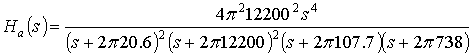where s is the complex variable.

### VBA Function

``` Function Aweighting(freq As Double) As Double
If NumberArg < 0 Then    ′ Evaluate argument.
Exit Function    ′ Exit to calling procedure.
Else
If freq > 0 Then
f2 = freq ^ 2```

` 			f4 = freq ^ 4`

``` 			′ Return A-weighting for this frequency.

Aweighting = 10 * Log(1.562339 * f4 / ((f2 + 107.65265 ^ 2) _
* (f2 + 737.86223 ^ 2))) / Log(10) _
+ 10 * Log(2.242881E+16 * f4 / ((f2 + 20.598997 ^ 2) ^ 2 _
* (f2 + 12194.22 ^ 2) ^ 2)) / Log(10)
Else
Aweighting = -1E+32
End If
End If
End Function
```